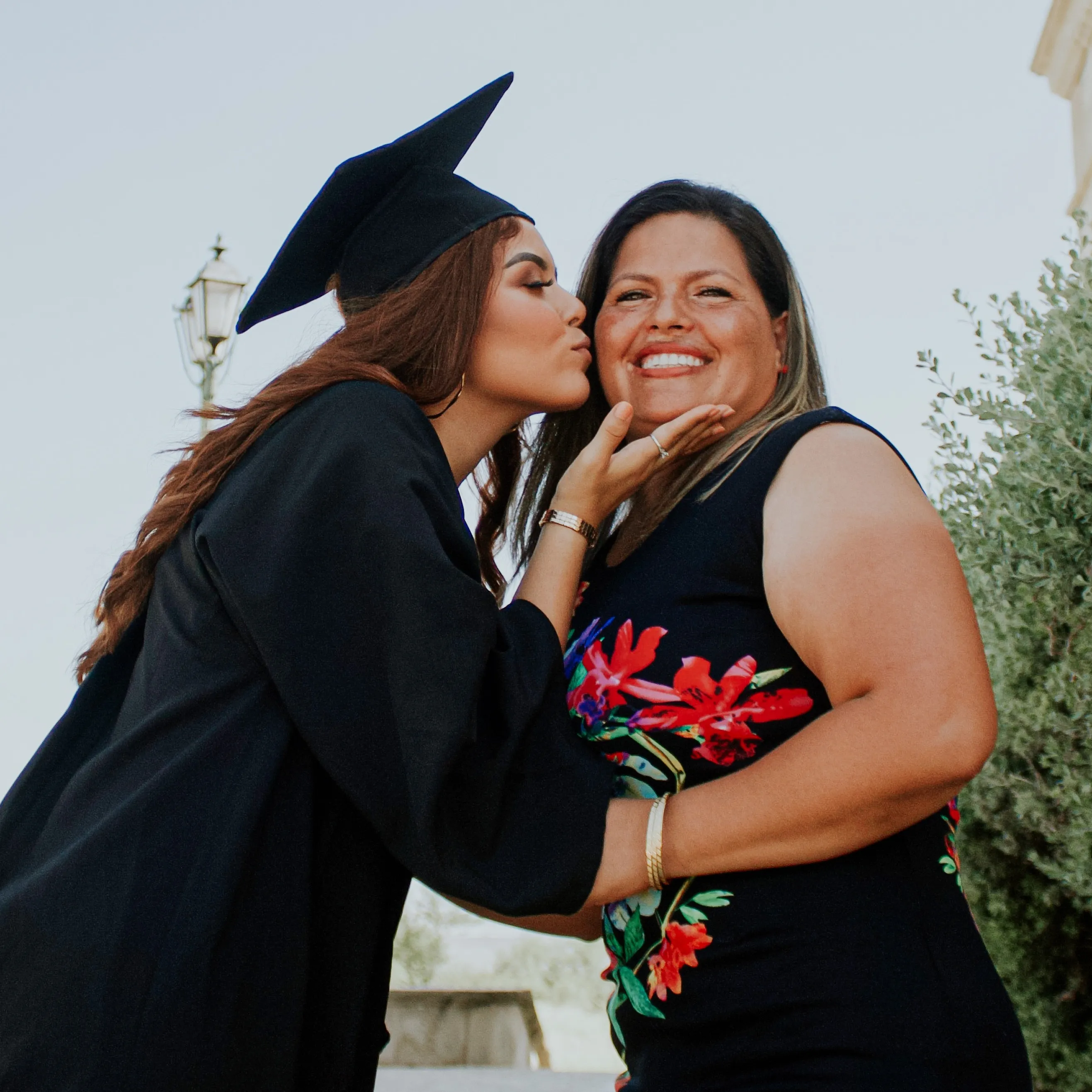I tutored mathematics for many years as I pursued my education, and I continue to tutor because I love it. I have a bachelor's degree in mathematics and statistics from UCSB and I tutor grades 6-College.

#### My tutoring style:

Instead of pulling information off of the textbook page, I prefer first to discuss ideas with students in a visual manner. From there we proceed to complete problem sets. Understanding the concepts are necessary for understanding the mechanics behind solving problems.

#### Success story:

I helped an Indonesian classmate of mine pass a class. This was important for her because her student visa would have been entered a complicated situation if she did not pass.

#### Hobbies and interests:

My hobbies include playing the piano, hiking, meditation, traveling, spending time with loved ones and reading (mostly fiction these days)

##### K-12 Mathematics
• Middle School Math
• Common Core Math
• Pre-Algebra
• Algebra I
• Algebra II
• Honors Algebra
• Geometry
• Trigonometry
• Pre-Calculus
• Calculus
• AP Calculus AB
• AP Calculus BC
• AP Statistics
##### College Mathematics
• College Algebra
• College Trigonometry
• College Calculus I
• College Calculus II
• College Calculus III
• Linear Algebra
• Statistics I
• Statistics II
• Finite Mathematics
• Differential Equations
• Formal Logic
• Combinatorics
• Number Theory
• Real Analysis

I helped an Indonesian classmate of mine pass a class. This was important for her because her student visa would have been entered a complicated situation if she did not pass.

Tutoring subjects:

Algebra
Trigonometry
Calculus
Differential Equations
Linear Algebra
Formal Logic
Number Theory
Real Analysis
Finite Mathematics